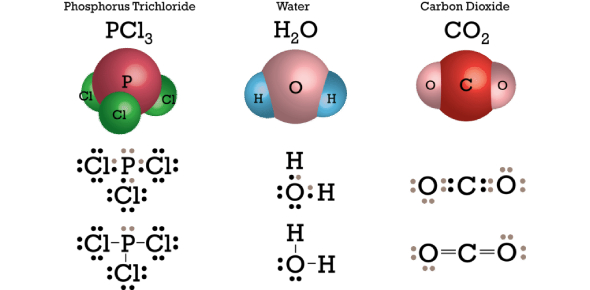# Covalent Bond Practice Test Quiz Questions!

10 Questions | Attempts: 10709SettingsCovalent bonds are produced when electrons are shared between atoms and are attracted by both atoms' nuclei. In pure covalent bonds, the electrons are divided equally. In polar covalent bonds, the electrons are distributed unequally, as one atom employs a greater force of magnetism on the electrons than the other. This quiz will help you to understand and know about covalent bonding. Good luck.

• 1.
What is  a chemical bond that involves sharing a pair of electrons between atoms in a molecule?
• A.

A cation

• B.

An ion

• C.

A covalent bond

• D.

An ionic bond

• 2.
Covalent chemical bonds where two lobes of one involved electron orbital overlap two lobes of the other is a
• A.

Ionic bond

• B.

Covalent bond

• C.

Sigma bond

• D.

Pi bond

• 3.
A chemical bond in which one atom loses an electron to form a positive ion and the other atom gains an electron to form a negative ion is a (an)
• A.

Cation

• B.

Ionic bond

• C.

Ionic bond

• D.

Covalent bond

• 4.
A chemical bond in which one atom loses an electron to form a positive ion and the other atom gains an electron to form a negative ion is
• A.

A sigma bond

• B.

An anion

• C.

Cation

• D.

Pi bond

• 5.
A positively charged ion
• A.

Sigma bond

• B.

Pi bond

• C.

Anion

• D.

Cation

• 6.
A negatively charged ion
• A.

A cation

• B.

An anion

• C.

A covalent bond

• D.

An ionic bond

• 7.
Electron-dot diagrams or Electron-dot structures
• A.

Lewis Dot Structure

• B.

An ion

• C.

Electronegativity

• D.

An anion

• 8.
Bonding occurs because of the attractions of
• A.

Ions

• B.

Neutrons

• C.

Electrons

• D.

Protons

• 9.
A bond in which a single pair electrons is shared between a pair of atoms is
• A.

A single bond

• B.

Double bond

• C.

Triple bond

• D.

Ionic bond

• 10.
A bond in which two pairs of electrons are shared between two atoms.
• A.

Triple bond

• B.

Double bound

• C.

Single bond

• D.

Ionic bond

## Related TopicsBack to top
×

Wait!
Here's an interesting quiz for you.